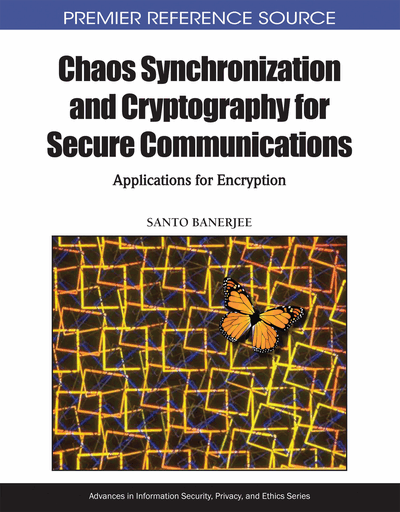# Encryption of Analog and Digital signals through Synchronized Chaotic Systems

Kehui Sun
DOI: 10.4018/978-1-61520-737-4.ch018
OnDemand:
(Individual Chapters)
Available
\$37.50
No Current Special Offers

## Abstract

Chaos is characterized by aperiodic, wideband, random-like, and ergodicity. Chaotic secure communication has become one of the hot topics in nonlinear dynamics since the early 1990s exploiting the technique of chaos synchronization. As distinguished by the type of information being carried, chaos-based communication systems can be categorized into analogy and digital, including four popular techniques such as Chaos Masking, Chaos Shift Keying, Chaos Modulation, and Chaos Spreading Spectrum. In this chapter, the principles of these schemes and their modifications are analyzed by theoretical analysis as well as dynamic simulation. In addition, chaos-based cryptography is a new approach to encrypt information. After analyzing the performances of chaotic sequence and designing an effective chaotic sequence generator, the authors briefly presented the principle of two classes of chaotic encryption schemes, chaotic sequence encryption and chaotic data stream encryption.
Chapter Preview
Top

## 1 Introduction

Chaos is an aperiodic long-term behavior in a deterministic system that exhibits sensitively dependent on initial conditions. There are three keywords in this definition, i.e. “aperiodic long-term behavior”, “deterministic” and “sensitive dependence on initial conditions”. “Aperiodic long-term behavior” means that the system’s trajectory in phase space does not settle down to any fixed points, periodic orbits, or quasi-periodic solutions as time tends to infinity. “Deterministic” means the irregular behavior of chaotic systems arises from intrinsic nonlinearities rather than noise. “Sensitive dependence on initial conditions” means that trajectories originating from very nearly identical initial conditions will diverge exponentially quickly.

Actually, two important features, sensitivity and randomness, give the way to application of secure communication. For a chaotic system, on one hand, the state space trajectories originating from two closely spaced initial conditions diverge exponentially. So its behaviors are unpredictable. It is useful for effective bits confusion and diffusion. Confusion and Diffusion are two principles in cryptology. “Confusion” is standing for substitution operations, and “diffusion” standing for transposition or permutation operations. These two principles are still actively used in modern ciphers. On the other hand, chaos is a kind of stochastic signals produced by deterministic model, so it has internal random, and has no relationship with external factors. It is useful for producing output signals with satisfactory statistics. This means the probability of symbols “1” and “0” for a chaotic sequence is equal.

As a kind of signal, chaos signals have some especial characters. For example, chaos signals are aperiodic, noise-like in time domain, and chaos signals are continuous and wideband in frequency domain. Chaos signals can be easily generated by deterministic nonlinear dynamic systems (ODE or iterative map). For chaos signals, its autocorrelation is impulse-like, delta function shown in Figure 1(a), and its cross-correlation is zero (low) shown in Figure 1(b).

Communication systems are designed primarily for conveying useful information from one place to another. Typical communication systems including amplitude modulated (AM), frequency modulated (FM), phase modulated (PM), cellular mobile communication and wireless local area networks. The simplest communication consists of only four components, namely an information source, a modulator, a channel and a demodulator, as represented by the function block diagram as shown in Figure 2 (Stavroulakis, 2005).

Since the late 1980s, chaos-based cryptography has attracted more and more attention from researchers in many different areas. It has been found that chaotic systems and cryptosystems share many similar properties. For instance, chaotic systems are sensitive to the initial conditions, which corresponds to the diffusion property of good cryptosystems (for a comparison of chaos and cryptography, see Table 1 (Alvarez & Li, 2006).

Table 1.
Comparison between chaos properties and cryptography properties
 Chaotic properties Cryptographic properties Description Ergodicity Confusion The output has the same distribution for any input. Sensitivity to initial conditions /control parameter Diffusion with a small change in the plaintext /secret key A small deviation in the input can cause a large change at the output. Mixing property Diffusion with a small change in one plain-block of the whole plaintext A small deviation in the local area can cause a large change in the whole space. Deterministic dynamics Deterministic pseudo-randomness A deterministic process can cause a random-like (pseudo-random) behavior. Structure complexity Algorithm (attack) complexity A simple process has a very high complexity.

## Complete Chapter List

Search this Book:
Reset